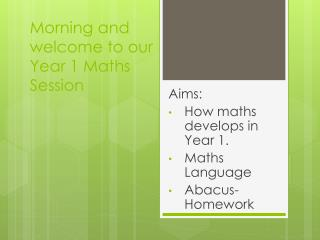Download PresentationMorning and welcome to our Year 1 Maths Session

# Morning and welcome to our Year 1 Maths Session - PowerPoint PPT Presentation

Morning and welcome to our Year 1 Maths Session. Aims: How maths develops in Year 1. Maths Language Abacus- Homework. What are your children aiming for?. Year 2 SATS. SAT type questions. Targets. Maths Dictionary. Useful websites. Expectations in Year 1. Mental Maths. Click HERE.I am the owner, or an agent authorized to act on behalf of the owner, of the copyrighted work described.
Download Presentation## Morning and welcome to our Year 1 Maths Session

Download Policy: Content on the Website is provided to you AS IS for your information and personal use and may not be sold / licensed / shared on other websites without getting consent from its author.While downloading, if for some reason you are not able to download a presentation, the publisher may have deleted the file from their server.

- - - - - - - - - - - - - - - - - - - - - - - - - - E N D - - - - - - - - - - - - - - - - - - - - - - - - - -
Presentation Transcript
1. Morning and welcome to our Year 1 Maths Session Aims: How maths develops in Year 1. Maths Language Abacus- Homework

2. What are your children aiming for? Year 2 SATS • SAT type questions. • Targets. • Maths Dictionary. • Useful websites.

3. Expectations in Year 1 Mental Maths • Click HERE The aim of mental maths should be for children wherever possible to do the calculation in their head. To do these children need to be given help ‘making pictures’ in their minds. Mental maths is not just about numbers but also about seeing shapes and being able to hold on to these and manipulate them in their head. Times tables and number facts are so important to learn ‘off by heart’ – this is known as mental recall. Facts should be recalled within 3 seconds.

4. Mathematical Vocabulary • Number Bonds e.g. 6 + 4 = 10 56 + 44 = 100 17 + 3 = 20 • Calculation or a number sentence. NOT a sum unless it’s an addition. • Times table and division facts e.g. 4 x 5 = 20 and 5 x 4 = 20 20 ÷ 4 = 5 and 20 ÷ 5 = 4 • Inverse e.g. 3 x 6 = 18 18 ÷ 6 = 3 64 – 22 = 42 42 + 22 = 64 • Place Value – Ones/Units, Tens, Hundreds, etc..

5. Odd and even numbers: Odd numbers end in 1, 3, 5, 7, 9 and even numbers end in 0, 2, 4, 6, 8. Partitioning: Splitting numbers to calculate and understand the value. e.g. 324 300 + 20 + 4 = 324 and 300 + 10 + 14 = 324 etc… Estimate: To make a logical guess based on previous knowledge and rounding. e.g. 96 x 3 = To estimate round 96 to the nearest 10 = 100 100 x 3 = 300, therefore the estimated answer would be 300. Rounding: Round down when the number ends in 1, 2, 3, 4 and up when it ends in 5, 6, 7, 8, 9.

6. Multiples: A number that is part of that times table e.g. 3 , 6, 9, 12… are all multiples of 3. Near doubles and doubles/halves. e.g. 9 + 8 = 17 Using knowledge of double 9 or double 8 to help you solve calculations. Double is the same as x2. Half is the same as ÷ 2. To x by 4 double and double again. To ÷ by 4 half and half again. RUCSAC – Read, Understand, Choose, Solve, Answer, Check. This is the process we follow to help the children understand word problems.

7. Vocabulary used for Problem Solving

8. Homework • Every week your child will be set homework online for Maths. • Please collect the handout that tells you how to log into Abacus. • Abacus • Teachers can access each account and it will tell them who has completed the set task.

9. Any Questions?